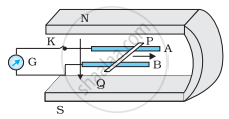# What is the Induced Emf in the Moving Rod If the Magnetic Field is Parallel to the Rails Instead of Being Perpendicular? - Physics

Numerical

Figure shows a metal rod PQ resting on the smooth rails AB and positioned between the poles of a permanent magnet. The rails, the rod, and the magnetic field are in three mutual perpendicular directions. A galvanometer G connects the rails through a switch K. Length of the rod = 15 cm, B = 0.50 T, resistance of the closed loop containing the rod = 9.0 mΩ. Assume the field to be uniform.

(a) Suppose K is open and the rod is moved with a speed of 12 cm s−1 in the direction shown. Give the polarity and magnitude of the induced emf.(b) Is there an excess charge built up at the ends of the rods when K is open? What if K is closed?

(c) With K open and the rod moving uniformly, there is no net force on the electrons in the rod PQ even though they do experience magnetic force due to the motion of the rod. Explain.

(d) What is the retarding force on the rod when K is closed?

(e) How much power is required (by an external agent) to keep the rod moving at the same speed = (12 cm s−1) when K is closed? How much power is required when K is open?

(f) How much power is dissipated as heat in the closed circuit? What is the source of this power?

(g) What is the induced emf in the moving rod if the magnetic field is parallel to the rails instead of being perpendicular?

#### Solution

Length of the rod, l = 15 cm = 0.15 m

Magnetic field strength, B = 0.50 T

Resistance of the closed loop, R = 9 mΩ = 9 × 10−3 Ω

(a) Induced emf = 9 mV; polarity of the induced emf is such that end P shows positive while end Q shows negative ends.

Speed of the rod, v = 12 cm/s = 0.12 m/s

Induced emf is given as:

e = Bvl

= 0.5 × 0.12 × 0.15

= 9 × 10−3 v

= 9 mV

The polarity of the induced emf is such that end P shows positive while end Q shows negative ends.

(b) Yes; when key K is closed, excess charge is maintained by the continuous flow of current.

When key K is open, there is excess charge built up at both ends of the rods.

When key K is closed, excess charge is maintained by the continuous flow of current.

(c) Magnetic force is cancelled by the electric force set-up due to the excess charge of opposite nature at both ends of the rod.

There is no net force on the electrons in rod PQ when key K is open and the rod is moving uniformly. This is because the magnetic force is cancelled by the electric force set-up due to the excess charge of opposite nature at both ends of the rods.

(d) Retarding force exerted on the rod, F = IBl

Where,

I = Current flowing through the rod

= "e"/"R" = (9 xx 10^-3)/(9 xx 10^-3) = 1 A

F = 1 × 0.5 × 0.15

= 75 × 10−3 N

(e) 9 mW; no power is expended when key K is open.

Speed of the rod, v = 12 cm/s = 0.12 m/s

Hence, power is given as:

P = Fv

= 75 × 10−3 × 0.12

= 9 × 10−3 W

= 9 mW

When key K is open, no power is expended.

(f) 9 mW; power is provided by an external agent.

Power dissipated as heat = I2 R

= (1)2 × 9 × 10−3

= 9 mW

The source of this power is an external agent.

(g) Zero

In this case, no emf is induced in the coil because the motion of the rod does not cut across the field lines.

Concept: Induced e.m.f. and Induced Current
Is there an error in this question or solution?

#### APPEARS IN

NCERT Physics Part 1 and 2 Class 12
Chapter 6 Electromagnetic Induction
Exercise | Q 6.14 | Page 231
NCERT Class 12 Physics Textbook
Chapter 6 Electromagnetic Induction
Exercise | Q 14 | Page 231

Share